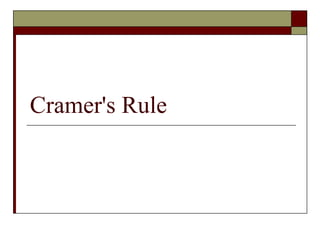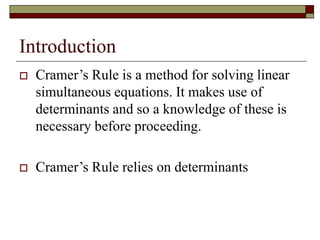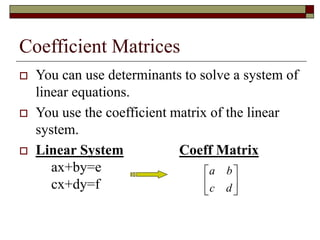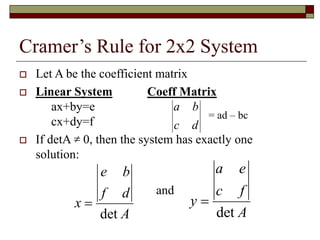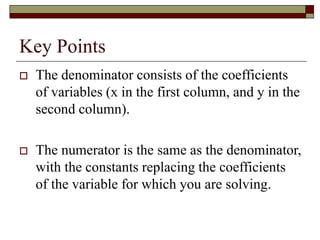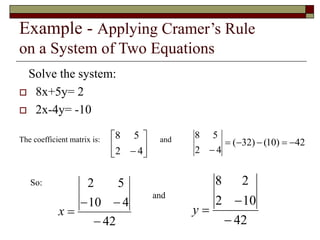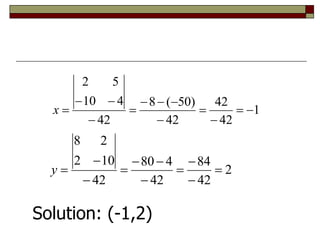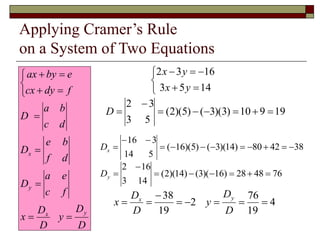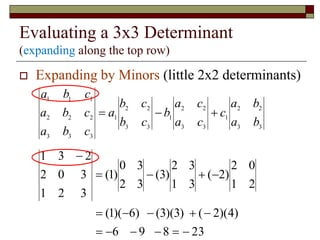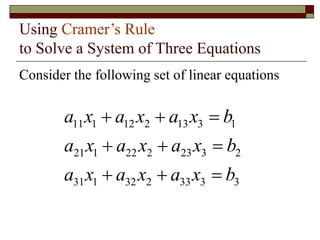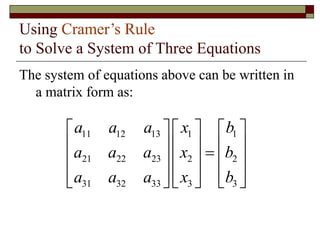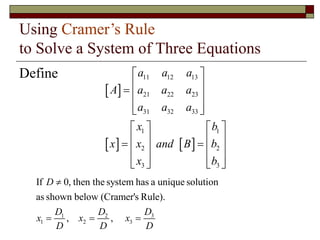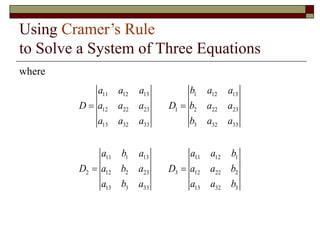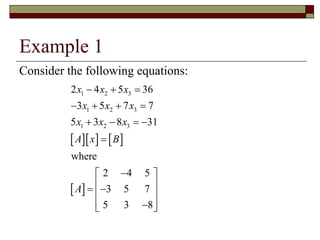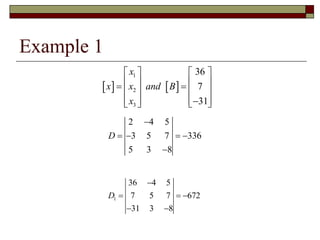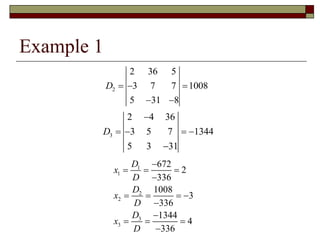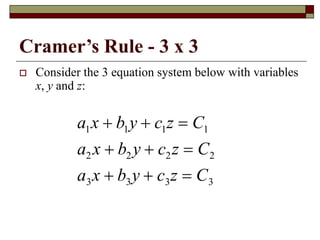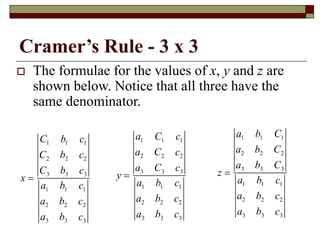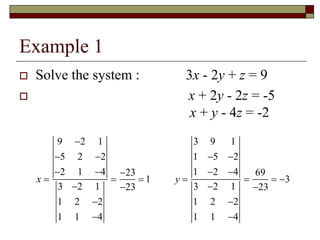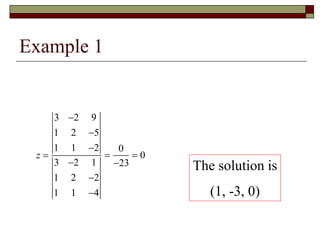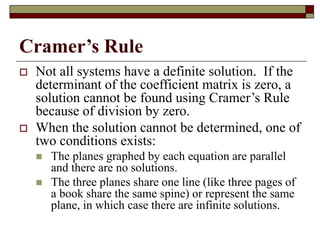1 of 21

### Cramer's Rule.ppt

1. Cramer's Rule
2. Introduction  Cramer’s Rule is a method for solving linear simultaneous equations. It makes use of determinants and so a knowledge of these is necessary before proceeding.  Cramer’s Rule relies on determinants
3. Coefficient Matrices  You can use determinants to solve a system of linear equations.  You use the coefficient matrix of the linear system.  Linear System Coeff Matrix ax+by=e cx+dy=f       d c b a
4. Cramer’s Rule for 2x2 System  Let A be the coefficient matrix  Linear System Coeff Matrix ax+by=e cx+dy=f  If detA 0, then the system has exactly one solution: and A d f b e x det  A f c e a y det   d c b a = ad – bc
5. Key Points  The denominator consists of the coefficients of variables (x in the first column, and y in the second column).  The numerator is the same as the denominator, with the constants replacing the coefficients of the variable for which you are solving.
6. Example - Applying Cramer’s Rule on a System of Two Equations Solve the system:  8x+5y= 2  2x-4y= -10 The coefficient matrix is: and So: and        4 2 5 8 42 ) 10 ( ) 32 ( 4 2 5 8       42 4 10 5 2     x 42 10 2 2 8    y
7. 1 42 42 42 ) 50 ( 8 42 4 10 5 2              x 2 42 84 42 4 80 42 10 2 2 8            y Solution: (-1,2)
8. Applying Cramer’s Rule on a System of Two Equations D D y D D x f c e a D d f b e D d c b a D f dy cx e by ax y x y x                     14 5 3 16 3 2 y x y x 19 9 10 ) 3 )( 3 ( ) 5 )( 2 ( 5 3 3 2         D 38 42 80 ) 14 )( 3 ( ) 5 )( 16 ( 5 14 3 16             x D 76 48 28 ) 16 )( 3 ( ) 14 )( 2 ( 14 3 16 2         y D 4 19 76 2 19 38         D D y D D x y x
9. Evaluating a 3x3 Determinant (expanding along the top row)  Expanding by Minors (little 2x2 determinants) 3 3 2 2 1 3 3 2 2 1 3 3 2 2 1 3 3 3 2 2 2 1 1 1 b a b a c c a c a b c b c b a c b a c b a c b a    23 8 9 6 ) 4 )( 2 ( ) 3 )( 3 ( ) 6 )( 1 ( 2 1 0 2 ) 2 ( 3 1 3 2 ) 3 ( 3 2 3 0 ) 1 ( 3 2 1 3 0 2 2 3 1                
10. Using Cramer’s Rule to Solve a System of Three Equations Consider the following set of linear equations 11 1 12 2 13 3 1 21 1 22 2 23 3 2 31 1 32 2 33 3 3 a x a x a x b a x a x a x b a x a x a x b         
11. Using Cramer’s Rule to Solve a System of Three Equations The system of equations above can be written in a matrix form as: 11 12 13 1 1 21 22 23 2 2 31 32 33 3 3 a a a x b a a a x b a a a x b                               
12. Using Cramer’s Rule to Solve a System of Three Equations Define       11 12 13 21 22 23 31 32 33 1 1 2 2 3 3 a a a A a a a a a a x b x x and B b x b                                    3 1 2 1 2 3 If 0, then the system has a unique solution as shown below (Cramer's Rule). , , D D D D x x x D D D                   
13. Using Cramer’s Rule to Solve a System of Three Equations where 11 12 13 1 12 13 12 22 23 1 2 22 23 13 32 33 3 32 33 11 1 13 11 12 1 2 12 2 23 3 12 22 2 13 3 33 13 32 3 a a a b a a D a a a D b a a a a a b a a a b a a a b D a b a D a a b a b a a a b      
14. Example 1 Consider the following equations:        1 2 3 1 2 3 1 2 3 2 4 5 36 3 5 7 7 5 3 8 31 where 2 4 5 3 5 7 5 3 8 x x x x x x x x x A x B A                          
15. Example 1     1 2 3 36 7 31 x x x and B x                          2 4 5 3 5 7 336 5 3 8 D       1 36 4 5 7 5 7 672 31 3 8 D      
16. Example 1 2 2 36 5 3 7 7 1008 5 31 8 D      3 2 4 36 3 5 7 1344 5 3 31 D       1 1 2 2 3 3 672 2 336 1008 3 336 1344 4 336 D x D D x D D x D               
17. Cramer’s Rule - 3 x 3  Consider the 3 equation system below with variables x, y and z: a1x  b1y  c1z  C1 a2 x  b2 y  c2z  C2 a3x  b3y  c3z  C3
18. Cramer’s Rule - 3 x 3  The formulae for the values of x, y and z are shown below. Notice that all three have the same denominator. x  C1 b1 c1 C2 b2 c2 C3 b3 c3 a1 b1 c1 a2 b2 c2 a3 b3 c3 y  a1 C1 c1 a2 C2 c2 a3 C3 c3 a1 b1 c1 a2 b2 c2 a3 b3 c3 z  a1 b1 C1 a2 b2 C2 a3 b3 C3 a1 b1 c1 a2 b2 c2 a3 b3 c3
19. Example 1  Solve the system : 3x - 2y + z = 9  x + 2y - 2z = -5 x + y - 4z = -2 x  9 2 1 5 2 2 2 1 4 3 2 1 1 2 2 1 1 4  23 23  1 y  3 9 1 1 5 2 1 2 4 3 2 1 1 2 2 1 1 4  69 23  3
20. Example 1 z  3 2 9 1 2 5 1 1 2 3 2 1 1 2 2 1 1 4  0 23  0 The solution is (1, -3, 0)
21. Cramer’s Rule  Not all systems have a definite solution. If the determinant of the coefficient matrix is zero, a solution cannot be found using Cramer’s Rule because of division by zero.  When the solution cannot be determined, one of two conditions exists:  The planes graphed by each equation are parallel and there are no solutions.  The three planes share one line (like three pages of a book share the same spine) or represent the same plane, in which case there are infinite solutions.